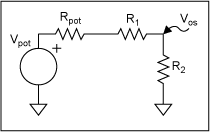# Finding the Thevenin Equivalent Circuit for the EPOT-Based Offset Resistor Network

### Abstract

This application note explains how to find the Thevenin equivalent circuit for an offset resistor network based on an electronically controlled potentiometer (EPOT).

To model the network shown below in Figure 1 as the Thevenin equivalent shown below in Figure 2, find the Thevenin Voltage (Vth) and the Thevenin Resistance (Rth). In this case, Vth is the same as VOS (with no external load present) and Rth is the resistance looking back from VOS with the voltage sources shorted out. Start by replacing the EPOT with its Thevenin equivalent and then substitute that replacement into the original network.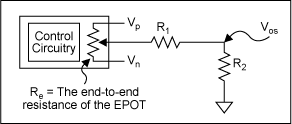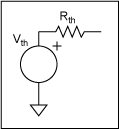Note 1: In this analysis, N = (Current EPOT setting)/(total number of taps). In other words, for a 32 tap EPOT, a setting of 8 makes N = 0.25.
Note 2: Vp = the positive supply voltage and Vn = the negative supply voltage
Note 3: Vpot = the voltage at the wiper of the EPOT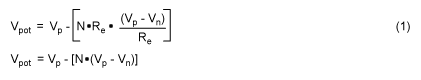To find the EPOT's equivalent resistance (Rpot), short out the sources, i.e. it's just the two resistances in parallel.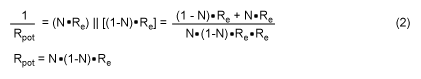The final step is to substitute the Thevenin circuit for the EPOT into the original circuit and find the overall Thevenin replacement.